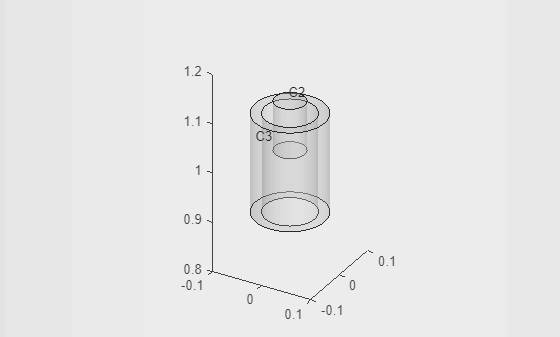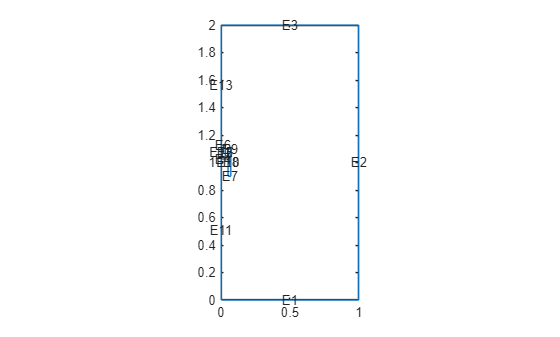# Magnetic Flux Density in Electromagnet

This example shows how to solve a 3-D magnetostatic problem for a solenoid with a finite length iron core. Using a ferromagnetic core with high permeability, such as an iron core, inside a solenoid increases magnetic field and flux density. In this example, you find the magnetic flux density for a geometry consisting of a coil with a finite length core in a cylindrical air domain.The first part of the example solves the magnetostatic problem using a 3-D model. The second part solves the same problem using an axisymmetric 2-D model to speed up computations.

### 3-D Model of Coil with Core

Create geometries consisting of three cylinders: a solid circular cylinder models the core, an annular circular cylinder models the coil, and a larger circular cylinder models the air around the coil.

```coreGm = multicylinder(0.03,0.1); coilGm = multicylinder([0.05 0.07],0.2,'Void',[1 0]); airGm = multicylinder(1,2);```

Position the core and coil so that the finite length core is located near the top of coil.

```coreGm = translate(coreGm,[0 0 1.025]); coilGm = translate(coilGm,[0 0 0.9]);```

Combine the geometries and plot the result.

```gm = addCell(airGm,coreGm); gm = addCell(gm,coilGm); pdegplot(gm,'FaceAlpha',0.2,'CellLabels','on')```Zoom in to see the cell labels on the core and coil.

```figure pdegplot(gm,'FaceAlpha',0.2,'CellLabels','on') axis([-0.1 0.1 -0.1 0.1 0.8 1.2])```Create an electromagnetic model and assign air geometry to the model.

```model3D = createpde('electromagnetic','magnetostatic'); model3D.Geometry = gm;```

Specify the vacuum permeability value in the SI system of units.

`model3D.VacuumPermeability = 1.2566370614E-6;`

Specify a relative permeability of 1 for all domains.

`electromagneticProperties(model3D,'RelativePermeability',1);`

Now specify the large relative permeability of the core.

```electromagneticProperties(model3D,'RelativePermeability',10000, ... 'Cell',2);```

Assign an excitation current using a function that defines counterclockwise current density in the coil.

```electromagneticSource(model3D,'CurrentDensity',@windingCurrent3D, ... 'Cell',3);```

Specify that the magnetic potential on the outer surface of the air domain is 0.

`electromagneticBC(model3D,'MagneticPotential',[0;0;0],'Face',1:3);`

Generate a mesh where only the core and coil regions are well refined and the air domain is relatively coarse to limit the size of the problem.

```internalFaces = cellFaces(model3D.Geometry,2:3); generateMesh(model3D,'Hface',{internalFaces,0.007});```

Solve the model.

`R = solve(model3D)`
```R = MagnetostaticResults with properties: MagneticPotential: [1×1 FEStruct] MagneticField: [1×1 FEStruct] MagneticFluxDensity: [1×1 FEStruct] Mesh: [1×1 FEMesh] ```

Find the magnitude of the flux density.

```Bmag = sqrt(R.MagneticFluxDensity.Bx.^2 + ... R.MagneticFluxDensity.By.^2 + ... R.MagneticFluxDensity.Bz.^2);```

Find the mesh elements belonging to the core and the coil.

`coreAndCoilElem = findElements(model3D.Mesh,'region','Cell',[2 3]);`

Plot the magnitude of the flux density on the core and coil.

```pdeplot3D(model3D.Mesh.Nodes, ... model3D.Mesh.Elements(:,coreAndCoilElem), ... 'ColorMapData',Bmag) axis([-0.1 0.1 -0.1 0.1 0.8 1.2])```Interpolate the flux to a grid covering the portion of the geometry near the core.

```x = -0.05:0.01:0.05; z = 1.02:0.01:1.14; y = x; [X,Y,Z] = meshgrid(x,y,z); intrpBcore = R.interpolateMagneticFlux(X,Y,Z); ```

Reshape `intrpBcore.Bx`, `intrpBcore.By`, and `intrpBcore.Bz` and plot the magnetic flux density as a vector plot.

```Bx = reshape(intrpBcore.Bx,size(X)); By = reshape(intrpBcore.By,size(Y)); Bz = reshape(intrpBcore.Bz,size(Z)); quiver3(X,Y,Z,Bx,By,Bz,'Color','r') hold on pdegplot(coreGm,'FaceAlpha',0.2);```### 2-D Axisymmetric Model of Coil with Core

Now, simplify this 3-D problem to 2-D using the symmetry around the axis of rotation.

First, create the geometry. The axisymmetric section consists of two small rectangular regions (the core and coil) located within a large rectangular region (air).

```R1 = [3,4,0.0,1,1,0.0,0,0,2,2]'; R2 = [3,4,0,0.03,0.03,0,1.025,1.025,1.125,1.125]'; R3 = [3,4,0.05,0.07,0.07,0.05,0.90,0.90,1.10,1.10]'; ns = char('R1','R2','R3'); sf = 'R1+R2+R3'; gdm = [R1, R2, R3]; g = decsg(gdm,sf,ns');```

Plot the geometry with the face labels.

`pdegplot(g,'FaceLabels','on')`Zoom in to see the face labels on the core and coil.

```figure pdegplot(g,'FaceLabels','on') axis([0 0.1 0.8 1.2])```Create an electromagnetic model for axisymmetric magnetostatic analysis and assign the geometry.

```model2D = createpde('electromagnetic','magnetostatic-axisymmetric'); geometryFromEdges(model2D,g);```

Specify the vacuum permeability value in the SI system of units.

`model2D.VacuumPermeability = 1.2566370614E-6;`

Specify a relative permeability of 1 for all domains.

`electromagneticProperties(model2D,'RelativePermeability',1);`

Now specify the large relative permeability of the core.

```electromagneticProperties(model2D,'RelativePermeability',10000, ... 'Face',3);```

Specify the current density in the coil. For an axisymmetric model, use the constant current value.

`electromagneticSource(model2D,'CurrentDensity',5E6,'Face',2);`

Assign zero magnetic potential on the outer edges of the air domain as the boundary condition.

`electromagneticBC(model2D,'MagneticPotential',0,'Edge',[2 8]);`

Generate a mesh.

`generateMesh(model2D,'Hmin',0.0004,'Hgrad',2,'Hmax',0.008);`

Solve the model.

`R = solve(model2D);`

Find the magnitude of the flux density.

```Bmag = sqrt(R.MagneticFluxDensity.Bx.^2 + ... R.MagneticFluxDensity.By.^2);```

Plot the magnitude of the flux density on the core and coil.

```pdeplot(model2D,'XYData',Bmag) xlim([0,0.05]); ylim([1.0,1.14])```Interpolate the flux to a grid covering the portion of the geometry near the core.

```x = 0:0.01:0.05; y = 1.02:0.01:1.14; [X,Y] = meshgrid(x,y); intrpBcore = R.interpolateMagneticFlux(X,Y);```

Reshape `intrpBcore.Bx` and `intrpBcore.By` and plot the magnetic flux density as a vector plot.

```Bx = reshape(intrpBcore.Bx,size(X)); By = reshape(intrpBcore.By,size(Y)); quiver(X,Y,Bx,By,'Color','r') hold on pdegplot(model2D); xlim([0,0.07]); ylim([1.0,1.14])```### Function Defining Current Density in Coil for 3-D Model

```function f3D = windingCurrent3D(region,~) [TH,~,~] = cart2pol(region.x,region.y,region.z); f3D = -5E6*[sin(TH); -cos(TH); zeros(size(TH))]; end```

### References

 Thierry Lubin, Kévin Berger, Abderrezak Rezzoug. "Inductance and Force Calculation for Axisymmetric Coil Systems Including an Iron Core of Finite Length." Progress In Electromagnetics Research B, EMW Publishing 41 (2012): 377-396. https://hal.archives-ouvertes.fr/hal-00711310.

## SupportGet trial now